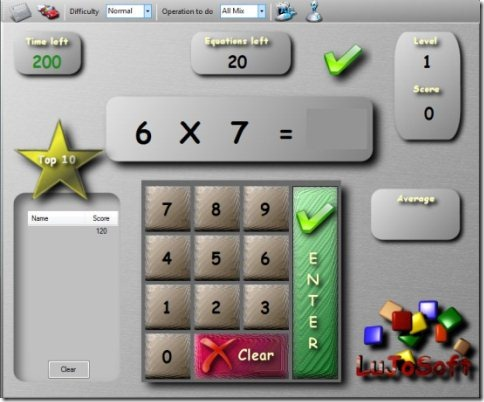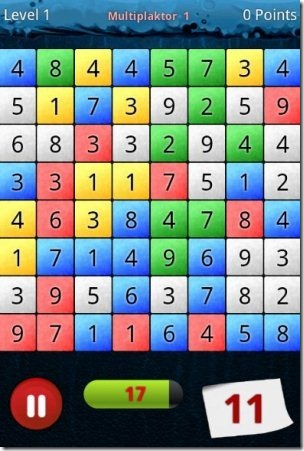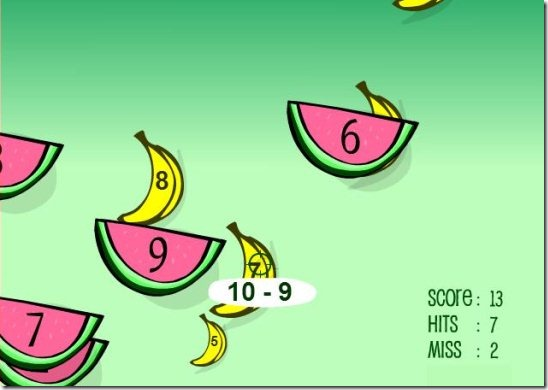Editor Ratings:
User Ratings:
[Total: 1 Average: 2]

Here is a list of 5 free games to learn Maths for kids. The list includes Math games that can be played on your PC, Android, and Online.

Maths is one of the best and useful subjects from our school days. Some kids find it easy and fun to learn Maths; but some do not. Some kids find it very boring that they neglect it because it gives more stress to brain. So, there are games which make learning Maths easy and fun too. So, try these games and learn Maths in a fun way and be a genius in Maths.

## Lujosoft KidsMath:Lujosoft KidsMath is a free math game for kids to learn Maths while playing on your PC. This game is specially designed for kids of age group 5 to 9.

Through this Math game kids can learn addition, subtraction, division, and multiplication. You can also choose the difficulty level between: easy, normal, difficult, and extreme. There are 20 equations at each level and the average score is also interpreted by the game. So, play these fun Math games against time to improve your speed and learn Maths in an interesting way.

## Bubble Sums:Bubble Sums is a free math learning software to learn Maths in a fun way. This game concentrates more on addition and subtraction.

Bubble Sums is easy to use and play. Just choose addition or subtraction, and use mix it up using your keyboard keys. Random questions will be flying in the form of bubbles and kids have to type in the answers using the numeric keys on the keyboard. If the answer is wrong you have to try again. This lightweight Math game can customized and played with both keyboard and mouse. Only limitation is that kids can learn only subtraction and addition with this game; but it is useful for kids who are starters to Maths.

## Math Maniac:Math Maniac is a free math game for Android. Now Android is also providing Math games for learning Maths in an easier way.

Math Maniac is an Android app which helps you learn Maths against time. There is a timer running and you have to complete the task within the time limit. If you are confused on how to play this game then go through the tutorial game; which teaches you how to play the game. This Android app is useful for learning Maths even while you are travelling or on the move using your Android phone.

## Math game:Math game is a free Android Math game to play and answer Math calculations. Every test in this Android game has a time limit of 60 seconds; so race against time to complete this Android math game.

In this math game you have to solve a simple math by combining the numbers in such a way that the result is equal to the number showed on the screen. You can also find different difficulty levels in quiz mode. Improve your math calculating ability with this free Android app and learn to solve mathematical calculations in a faster way.

## Sheppard Software:Sheppard Software is a website where your kids can learn Maths by playing simple games. Play games of your choice as there are many games available in this site.

Sheppard software math games are divided into categories like: basic operations, mixed operations, fractions, decimals, place value, telling time, money, early math, geometry, measurement, integers, and round estimate. Each category has number of games included in it. So, choose according to the syllabus of the kid and play to learn Maths while playing games. This game offers both relaxed mode and timer mode you have to shoot the answers with mouse and the question is displayed below your mouse. Play these simple games according to categories you need and have fun learning Math.